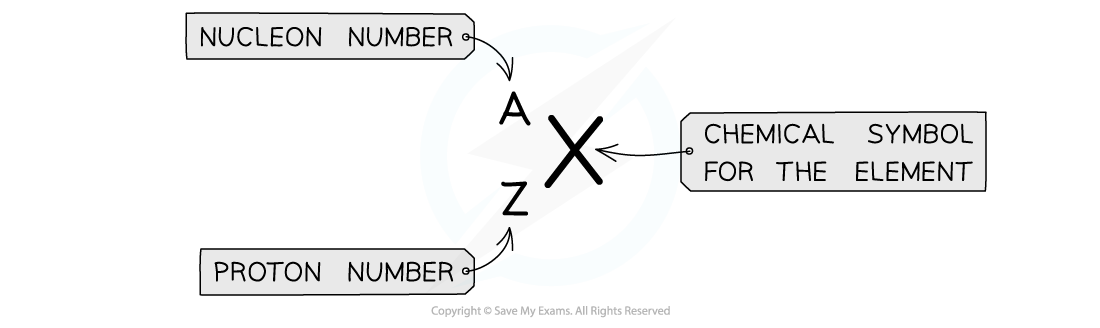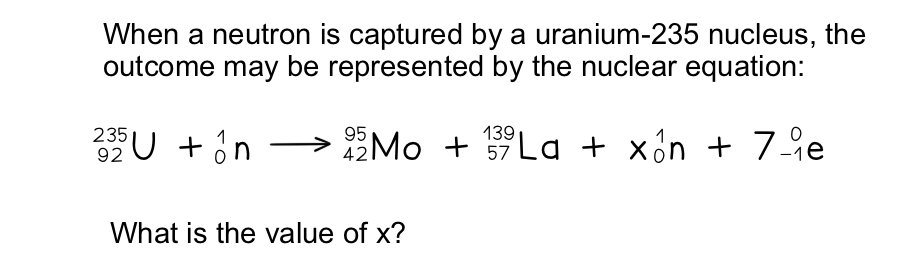# CIE A Level Physics复习笔记23.1.2 Nuclear Equations

### Representing Simple Nuclear Reactions

• Nuclear reactions can be represented by balanced equations of nuclei in the AZX formAZX notation for atomic nuclei

• The top number A represents the nucleon number or the mass number
• Nucleon number (A) = total number of protons and neutrons in the nucleus
• The lower number Z represents the proton or atomic number
• Proton number (Z) = total number of protons in the nucleus

#### Worked ExampleStep 1:            Balance the nucleon numbers (the top number)

235 + 1 = 95 + 139 + x(1) + 7(0)

Step 2:            Rearrange to find the value of x

x = 235 + 1 − 95 − 139 = 2